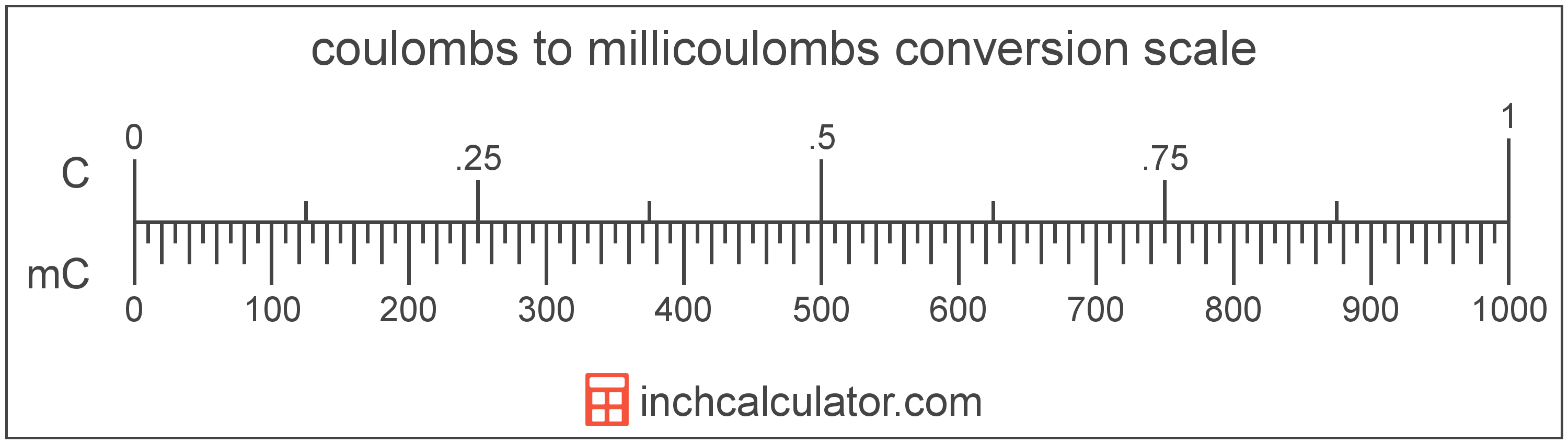# Millicoulombs to Coulombs Conversion

Enter the electric charge in millicoulombs below to get the value converted to coulombs.

Results in Coulombs:1 mC = 0.001 C

## How to Convert Millicoulombs to CoulombsTo convert a millicoulomb measurement to a coulomb measurement, divide the electric charge by the conversion ratio.

Since one coulomb is equal to 1,000 millicoulombs, you can use this simple formula to convert:

coulombs = millicoulombs ÷ 1,000

The electric charge in coulombs is equal to the millicoulombs divided by 1,000.

For example, here's how to convert 5,000 millicoulombs to coulombs using the formula above.
5,000 mC = (5,000 ÷ 1,000) = 5 C

## Millicoulombs

The millicoulomb is 1/1,000 of a coulomb, which is the electric charge equal to one ampere of current over one second.

The millicoulomb is a multiple of the coulomb, which is the SI derived unit for electric charge. In the metric system, "milli" is the prefix for 10-3. Millicoulombs can be abbreviated as mC; for example, 1 millicoulomb can be written as 1 mC.

## Coulombs

One coulomb is the electric charge equal to one ampere of current over one second.

The coulomb can be expressed as QC = IA × ts

The charge in coulombs is equal to the current in amperes times the time in seconds.

The coulomb is the SI derived unit for electric charge in the metric system. Coulombs can be abbreviated as C; for example, 1 coulomb can be written as 1 C.

## Millicoulomb to Coulomb Conversion Table

Millicoulomb measurements converted to coulombs
Millicoulombs Coulombs
1 mC 0.001 C
2 mC 0.002 C
3 mC 0.003 C
4 mC 0.004 C
5 mC 0.005 C
6 mC 0.006 C
7 mC 0.007 C
8 mC 0.008 C
9 mC 0.009 C
10 mC 0.01 C
20 mC 0.02 C
30 mC 0.03 C
40 mC 0.04 C
50 mC 0.05 C
60 mC 0.06 C
70 mC 0.07 C
80 mC 0.08 C
90 mC 0.09 C
100 mC 0.1 C
200 mC 0.2 C
300 mC 0.3 C
400 mC 0.4 C
500 mC 0.5 C
600 mC 0.6 C
700 mC 0.7 C
800 mC 0.8 C
900 mC 0.9 C
1,000 mC 1 C

## References

1. International Bureau of Weights and Measures, The International System of Units, 9th Edition, 2019, https://www.bipm.org/documents/20126/41483022/SI-Brochure-9-EN.pdf